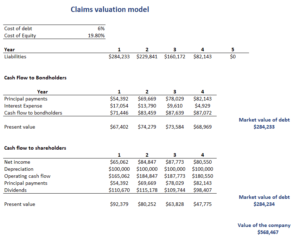# Claims valuation approach

The claims valuation approach is a method to analyze a company’s income such that it can be used to value the company. In particular, the claims valuation method divides operating cash flows based on the claims of debt and equity holders. These debt and equity cash flows can then be valued separately and added together to determine the value of the company.

The method uses the notion that that assets must equal liabilities plus equity. This implies that every asset on a company’s balance sheet must be financed by a combination of equity and debt.

On this page, we discuss the claims valuation model and illustrate how to apply it using a numerical example. The Excel spreadsheet used to create the example is available for download at the bottom of the page.

## Claims valuation approach definition

The claims valuation approach separates the cash flows provided by the asset into the proportionate debt and equity components:

• The cash flows to debt holders is the interest and principal payments and are discounted at the cost of debt
• The cash flows to equity holders are dividends and share repurchases and are discounted at the cost equity.

The sum of the present value of both components is the value of the company. Let’s have a look at an example.

## Claims valuation approach example

Let’s consider an example to illustrate the method. The following table illustrates how we can determine the cash flows to the debt holders and equity holders separately. The present value of these cash flows are then the value of the companyThe spreadsheet used to create the table is available at the bottom of the page.

## Summary

We discussed the claims valuation model. This method can be used to divide the cash flows an assets generates. Discounting both streams separately at the appropriate discount rate and summing them will yield the value of the company.

### Download the Excel spreadsheet

Want to have an implementation in Excel? Download the Excel file: Claims valuation model